### Home > AC > Chapter 14 > Lesson 14.3.1.3 > Problem3-43

3-43.
1. Solve the following systems of equations. Homework Help ✎

1. 3x − 2y = 14
−2x + 2y = − 10

2. y = 5x + 3
−2x − 4y = 10

3. Which system above is most efficiently solved by using the Substitution Method? Explain.

4. Which system above is most efficiently solved by using the Elimination Method? Explain.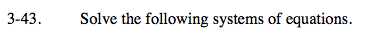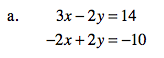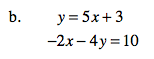If you need a reminder of how to solve systems of equations, refer to the Math Notes boxes in Lessons 2.1.1 and 2.1.3.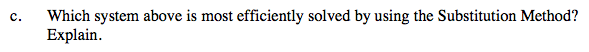Which criteria make a system easier to solve with the Substitution Method?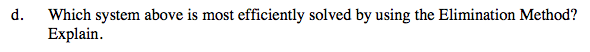Which criteria make a system easier to solve with the Elimination Method?